Friday, September 22, 2023

# Bridge Rectifier Circuit, Construction, Working, and Types

By Venus Kohli

A Rectifier is an electronic circuit that converts an input AC voltage into a DC voltage at the output terminal. This output is known as Rectified output voltage. Rectifiers are mainly used in power supply, providing DC voltage for electronic devices to work.

Rectifiers are categorized into two types depending on the operation-

• Half Wave Rectifiers
• Full Wave Rectifiers

We have already understood the operation of Half Wave Rectifiers and Full Wave Rectifiers.

The Half Wave Rectifier converts AC input voltage into output DC voltage by conducting in either one of the positive or negative half cycles. As a result, it passes one cycle and blocks the other.

Since one of the cycles passes the circuit and another gets blocked, one-half cycle is always wasted. This wastage leads to loss of power and poor efficiency by producing a low output voltage.

To make the process of rectification more efficient, Full Wave Rectifiers have proven to be effective. Full Wave Rectifiers utilize both positive and negative half cycles of the input voltage to produce an output voltage.

There are two types of full wave rectifiers-

• Bridge Full Wave Rectifier
• Centre Tap Full Wave Rectifier

## Bridge Rectifier

Bridge Rectifier is a type of Full Wave Rectifier that uses four diodes to form a close-loop bridge. The diodes conduct in pairs through each positive and negative half cycle, leading to no wastage of power.

Bridge Rectifier does not require a center tap over the secondary winding of the transformer. The input is fed through a transformer to the diagonal of the diode bridge. The transformer of this circuit is always busy because it supplies power all the time in both cycles of input AC, unlike the center tap rectifier that uses 50% of the transformer.

Bridge Rectifier comes in various types-

• Single Phase and Three Phase Bridge Rectifier
• Uncontrolled Bridge Rectifier
• Controlled Bridge Rectifier

## Single Phase Uncontrolled Bridge Rectifier

### Construction:

A single Phase Bridge Rectifier is constructed using four Diodes D1, D2, D3, and D4, connected in a closed loop configuration that forms a bridge. The diodes are arranged in a manner that they conduct in pairs during positive half cycles.

The input AC voltage is applied through a transformer across the Diagonal C of the bridge. The load resistor RL is connected between Diagonal C and D.

The output rectified DC voltage is obtained across the load from Diagonal D.

### Operation:

#### Positive Half Cycle:

During the positive half cycle of the input AC supply (0 to π),

The polarity of the secondary voltage across terminal A is positive with respect to terminal B.

This causes the Diodes D1 and D2 to be forward-biased and Diodes D3 and D4 to be reversed-biased.

D1 and D2 diodes create a path of short circuits and start conducting while diodes D3 and D4 behave as open circuits. The load current starts to flow through the short circuit path created by both D1, and D2 diodes. The direction of the load current is from D1, RL, to D2. The voltage across load resistor RL is positive at terminal D and negative at terminal C.

#### Negative Half Cycle:

During the negative half cycle of the input AC supply (π to 2π),

The polarity of the secondary voltage across terminal B is positive with respect to terminal A.

D3 and D4 diodes create a path of short-circuit and start conducting while diodes D1 and D2 behave as open circuits. The load current starts to flow through the short circuit path created by both D3, and D4 diodes. The direction of the load current is from D3, RL, to D4. The voltage across load resistor RL is positive at terminal D and negative at terminal C.

It has to be noted that both the pair of Diodes D1, D2, D3, and D4 conduct in half cycles alternately. These pairs of diodes don’t conduct at the same time.

In both cycles, the load current flows through the positive half cycles of the input AC voltage and in the same direction through the load resistor RL. The polarity of the voltage across RL is the same as the direction of load current through conducting states of diodes D1, D2, and D3, D4. The output pulse can either be completely positive or negative.

Here, the output pulse obtained is positive. This unidirectional current means DC. Hence, the input AC voltage is converted into DC voltage at the output.

The obtained output rectified pulse is pulsating in nature. To purify this, we have to place a filter capacitor at the output to get a pure DC voltage.

### Few Parameters of a Single-Phase Bridge Rectifier

The Characteristics of a bridge Rectifier include the following-

• Efficiency
• Peak Inverse Voltage
• Ripple Factor

#### 1.) The efficiency of a Bridge Rectifier:

The efficiency of a bridge rectifier is defined as the ability of the bridge rectifier to convert input Alternating Current (AC) to Direct Current (DC). Simply, it is the ratio between Output DC power and Input AC power.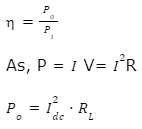Firstly, we need to calculate Idc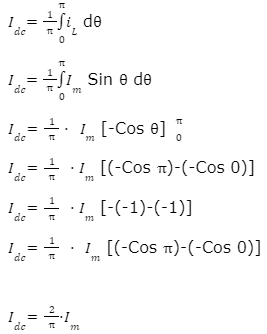So, the equation of output power becomes,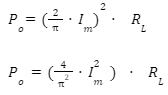We know,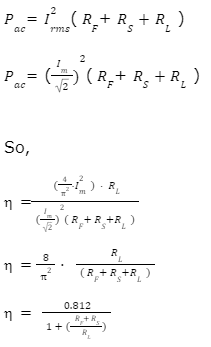The value for RF+RS is very very less than RL.

Hence, the negligible value can be ignored.

η = 0.812

η(in percentage)= 81.2%

#### 2.) Peak Inverse Voltage:

Peak Inverse Voltage is the maximum Voltage the rectifier can attain in reverse bias without damaging itself. The Peak Inverse Voltage for Bridge Rectifier is Vm.

The Peak Inverse Voltage of a Bridge Rectifier is half of a Centre Tap Rectifier

#### 3.) Ripple Factor:

The output of a Bridge rectifier is not pure DC, but rather pulsating DC as it contains a mixture of DC and AC. The AC components in the rectified output are called ripples.

Ripple Factor is defined as the percentage of ac component (or ripples) in the rectified DC output. Ripple Factor should be as low as possible. To reduce this factor, we use a filter capacitor at the output.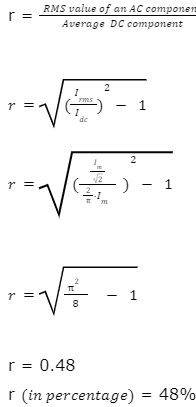## Three-Phase Uncontrolled Full Wave Bridge Rectifier

The 3-phase uncontrolled bridge rectifier is a network of 6 diodes that conduct in pairs for each cycle. A network of four diodes with two phases operates as a single-phase uncontrolled bridge rectifier.

The Diodes conduct in matching pairs as they form a series connections with current flowing through them. The two diodes belonging to the different phases conduct themselves together. This is because a single phase has positive and negative peak values at the same time.

### Construction:

The input AC voltage is fed from a 3-phase delta star Q3 transformer.

The positive terminal of the load is connected to the cathode of Diodes D1, D3, and D5. The anode of Diodes D1, D3, and D5 are connected to the input supply.

Similarly, the negative terminal of the load is connected to the anode of Diodes D2, D4, and D6. The cathode of Diodes D2, D4, and D6 are connected to the input supply.

Diodes D1, D3, and D5 form a group of diodes that feed to the positive terminal.

Diodes D2, D4, and D6 form a group of diodes that feed to the positive terminal.

### Connections:

• The cathode of Diode D2 and the anode of Diode D1 are connected to Phase R.
• The cathode of Diode D4 and the anode of Diode D3 are connected to Phase Y.
• The cathode of Diode D6 and the anode of Diode D5 are connected to Phase B.

Each input secondary terminal is connected to the cathode of one diode and the anode of the other to form a phase.

### Phase Formation:

• Diodes D1, D2, D3, and D4 form a phase network with R and Y of the input secondary star terminal.
• Diodes D1, D2, D5, and D6 form a phase network with R and B of the input secondary star terminal.
• Diodes D3, D4, D5, and D6 form a phase network with Y and B of the input secondary star terminal.

The load resistor RL is a part of every bridge network formed.

The two pairs of Diodes in a phase do not conduct at the same time because each phase has negative and positive peaks at the same time.

Each phase is separated by π/3 or 60°, meaning that the conduction angle of the Diode-Pair for a single cycle is π/3 or 60°. Hence, each diode conducts for 2π/3 or 120° or in a single cycle.

### Operation:

Case 1:

In the phase network of R and Y,

D1, D2, D3, and D4 form a Bridge rectifier.

When VB=0

VR reaches the maximum positive peak value and VY reaches the maximum negative peak value.

This causes Diodes D1 and D4 forward bias and Diodes D2 and D3 reverse bias.

The Diodes D2 and D3 act as open circuits.

The Diodes D1 and D4 form a series connection with RL and start conducting.

The current flows from D1 to RL and D4.

Hence, we obtain a positive pulse for voltage across this bridge network: VRY.

Case 2:

In the phase network of R and B,

D1, D2, D5, and D6 form a Bridge rectifier.

When VY=0

VR reaches the maximum positive peak value and VB reaches the maximum negative peak value.

This causes Diodes D1 and D6 forward bias and Diodes D2 and D5 reverse bias.

The Diodes D2 and D5 act as open-circuit.

The Diodes D1 and D6 form a series connection with RL and start conducting.

The current flows from D1 to RL and D6.

Hence, we obtain a positive pulse for voltage across this bridge network: VRB.

Case 3:

In the phase network of Y and B,

D3, D4, D5, and D6 form a Bridge rectifier.

When VR=0

VY reaches the maximum positive peak value and VB reaches the maximum negative peak value.

This causes Diodes D3 and D6 forward bias and Diodes D4 and D5 reverse bias.

The Diodes D4 and D5 act as an open circuit.

The Diodes D3 and D6 form a series connection with RL and start conducting.

The current flows from D3 to RL and D6.

Hence, we obtain a positive pulse for voltage across this bridge network: VYB.

Case 4:

In the phase network of Y and R,

D1, D2, D3, and D4 form a Bridge rectifier.

When VB=0

VY reaches the maximum positive peak value and VR reaches the maximum negative peak value.

This causes Diodes D3 and D2 forward bias and Diodes D1 and D4 reverse bias.

The Diodes D1 and D4 act as open circuit.

The Diodes D3 and D2 form a series connection with RL and start conducting.

The current flows from D3 to RL and D2.

Hence, we obtain a positive pulse for voltage across this bridge network: VYR.

Case 5:

In the phase network of B and R,

D1, D2, D5, and D6 form a Bridge rectifier.

When VY=0

VB reaches the maximum positive peak value and VR reaches the maximum negative peak value.

This causes Diodes D5 and D2 forward bias and Diodes D1 and D6 reverse bias.

The Diodes D1 and D6 act as open circuit.

The Diodes D5 and D2 form a series connection with RL and start conducting.

The current flows from D5 to RL and D2.

Hence, we obtain a positive pulse for voltage across this bridge network: VBR.

Case 6:

In the phase network of B and Y,

D3, D4, D5, and D6 form a Bridge rectifier.

When VR=0

VB reaches the maximum positive peak value and VY gets the maximum negative peak value.

This causes Diodes D5 and D4 forward bias and Diodes D3 and D6 reverse bias.

The Diodes D4 and D6 act as open circuit.

The Diodes D5 and D4 form a series connection with RL and start conducting.

The current flows from D5 to RL and D4.

Hence, we obtain a positive pulse for voltage across this bridge network: VBY.

#### Waveform:

We hope now you are well-informed about the Bridge Rectifiers. Still, if you have any doubt, please feel free to ask in the comment section below, or you can use our Forum to connect with our Engineers.